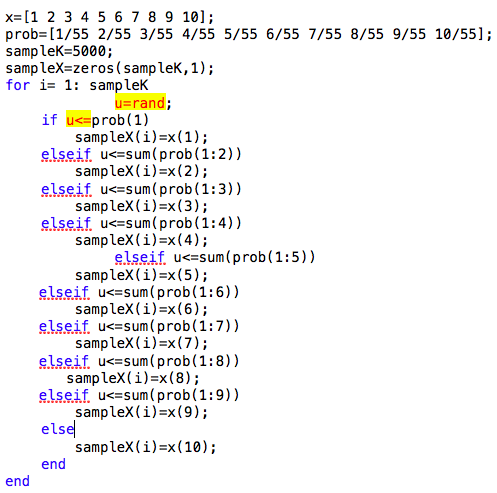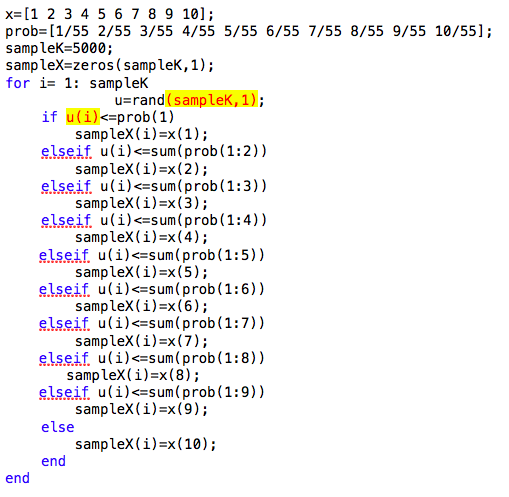# Generate n samples of random variables based on a given discrete uniform distributions. Can anyone tell me what is the difference between the codes I wrote?

15 views (last 30 days)
DDD on 8 Sep 2020
Commented: Rena Berman on 9 Oct 2020 at 16:39
Question: Write a MATLAB program to sample K values from the probability mass function Pi=i/55, i=1, 2, ….10. Plot the histogram of generated values for K=50, 500, and 5000.
Version 1:Version 2:I am new to Matlab. I don't know the difference, but both codes get the same results.

Rik on 11 Sep 2020
Why did you delete your question? That is very rude.
Rena Berman on 9 Oct 2020 at 16:39

Walter Roberson on 8 Sep 2020
The first code generates one new random number each time through the loop, for a total of sampleK random numbers generated out of which sampleK random numbers are used.
The second code generates sampleK random numbers each time through the loop, and uses the i'th of them, ignorning the others, for a total of sampleK * sampleK random numbers generated out of which sampleK random numbers are used.
If you were to move the
u = rand(sampleK,1);
to before the for loop, then you would be making one call that generated sampleK random numbers all at one time, and then used one at a time. That would be more efficient than generating one random number sampleK times.

Show 1 older comment
James Tursa on 8 Sep 2020
You might want to look at the result of cumsum(prob) instead of doing all the sum(prob(1:2)) etc. calculations individually.
Walter Roberson on 8 Sep 2020
DDD on 8 Sep 2020
Honestly, when I saw this question, my first instinct was to solve it using randsample().
The following is what I did, but I am required to solve this question using a uniform random number generator.
Deleted